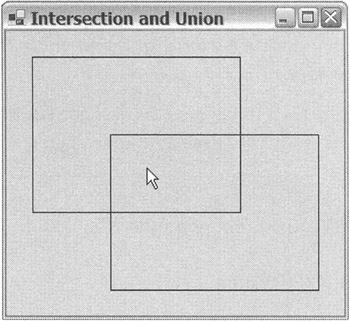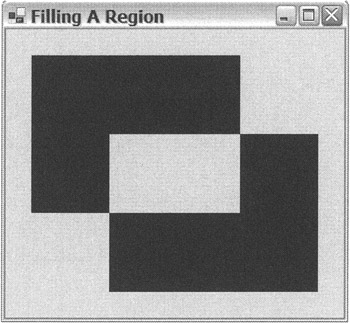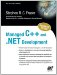# Common Utility Structures

When you render your own text, shape, or image, you need to be able to tell the Graphics class where to place it and how big it is. It is not surprising that the .NET Framework class library provides a small assortment of structures and a class to do just that. Here they are in brief:

• Point/PointF is used to specify location.

• Size/SizeF is used to specify size.

• Rectangle/RectangleF is used to specify both location and size at the same time.

• Region is used to specify combinations of rectangles and regions.

All of these types use units of measure configured by the property PageUnit within the Graphics class. You need to take care that you always configure PageUnit consistently, or you might find that even though the same values are placed in these structures, they in fact represent different locations and sizes.

All the structures have Int32 and Single versions. Both provide the same functionality. The only real difference is the level of granularity that is supported in numeric values stored within the structures. In most cases, the Int32 version will be good enough, but if you want finer granularity, you might want to choose the Single version. Just remember that ultimately, the resolution of the drawing surface will decide how the shape, image, or text is displayed.

### Point and PointF

As the name of this structure suggests, Point/PointF is an (x, y) location in units. Remember that units do not necessarily mean pixels. Pixels are only the default. The Point/PointF structure provides a few members (see Table 11-5) to aid in their manipulation.

Table 11-5: Common Point/PointF Members

MEMBER

DESCRIPTION

+ operator

Translates a Point/PointF by a Size/SizeF.

- operator

Translate a Point/PointF by the negative of a Size/SizeF.

== operator

Compares the equality of two points. Both Xs and Ys must equal for the point to equal.

! = operator

Compares the inequality of two points. If either the Xs or Ys don't equal, then the points don't equal.

IsEmpty

Specifies if the point is empty.

Ceiling()

Static member that returns next higher integer Point from a PointF.

Offset()

Translates the point by the specified x and y amounts.

Round()

Static member that returns a rounded Point from a PointF.

Truncate()

Static member that returns a truncated Point from a PointF.

X

Specifies the x coordinate of the point.

Y

Specifies the y coordinate of the point.

To access the X or Y values within the Point/PointF structure, you simply need to access the X or Y property:

` Drawing::Point a = Drawing::Point(10,15); Int32 x = a.X; Int32 y = a.Y; `

Casting from Point to PointF is implicit, but to convert from PointF, you need to use one of two static methods: Round() or Truncate(). The Round() method rounds to the nearest integer, and the Truncate() method simply truncates the number to just its integer value.

` Drawing::Point  a = Drawing::Point(10,15); Drawing::PointF b = a; Drawing::Point  c = Drawing::Point::Round(b); Drawing::Point  d = Drawing::Point::Truncate(b); `

The Offset() method is only found in Point, and it translates the point by the x and y coordinates passed to it.

` a.Offset(2, -3); `

The method is cumbersome as it returns void. I think it should return a Point type. I think it should also be a member of PointF.

### Size and SizeF

Mathematically, Size/SizeF and Point/PointF are virtually the same. How they differ is really just conceptually. Point/PointF specifies where something is, whereas Size/SizeF specifies how big it is. Point/PointF and Size/SizeF even have many of the same members (see Table 11-6). The biggest difference is that sizes have widths and heights, whereas the points have x and y coordinates.

Table 11-6: Common Size/SizeF Members

MEMBER

DESCRIPTION

+ operator

- operator

Subtracts one size from another.

== operator

Compares the equality of two sizes. Both Widths and Heights must equal for the points to equal.

! = operator

Compares the inequality of two sizes. If either Widths or Heights don't equal, then the points don't equal.

IsEmpty

Specifies if the size is empty.

Ceiling()

Static member that returns the next higher integer Size from a SizeF.

Round()

Static member that returns a rounded Size from a SizeF.

Truncate()

Static member that returns a truncated Size from a SizeF.

Height

Specifies the height of the size.

Width

Specifies the width of the size.

It is possible to add or subtract two sizes and get a size in return. It is also possible to subtract a size from a point that returns another point. Adding or subtracting points generates a compiler error.

` Drawing::Size sizeA = Drawing::Size(100, 100); Drawing::Size sizeB = Drawing::Size(50, 50); Drawing::Size sizeC = sizeA + sizeB; Drawing::Size sizeD = sizeC - sizeB; Drawing::Point pointA = Drawing::Point(10, 10) + sizeD; Drawing::Point pointB = pointA - sizeC; `

You can cast Point/PointF to Size/SizeF. What happens is the value of X becomes Width and the value of Y becomes Height and vice versa. The following code shows how to implement all the combinations. It also shows the Size to SizeF combinations:

` size   = point; point  = size; sizeF  = pointF; pointF = (Drawing::PointF)sizeF; sizeF  = (Drawing::Size)point; pointF = (Drawing::Point)size; sizeF  = size; size  = Drawing::Size::Round(pointF); size  = Drawing::Size::Truncate(pointF); point = Drawing::Point::Round((Drawing::PointF)sizeF); point = Drawing::Point::Truncate((Drawing::PointF)sizeF); size  = Drawing::Size::Round(sizeF); size  = Drawing::Size::Truncate(sizeF); `

### Rectangle and RectangleF

As I'm sure you can guess, the Rectangle/RectangleF structure represents the information that makes up a rectangle. It's really nothing more than a combination of a Point structure and a Size structure. The Point specifies the starting upper-left corner and the Size specifies the size of the enclosed rectangular area starting at the point. There is, in fact, a Rectangle/RectangleF constructor that takes as its parameters a Point and a Size.

The Rectangle structure provides many properties and methods (see Table 11-7), a few of which are redundant. For example, there are properties called Top and Left that return the exact same thing as the properties X and Y.

Table 11-7: Common Rectangle/RectangleF Members

MEMBER

DESCRIPTION

= =

Returns whether the rectangle has the same location and size

! =

Returns whether the rectangle has different location or size

Bottom

Returns the y coordinate of the bottom edge

Ceiling()

Static member that returns the next higher integer Rectangle from a RectangleF

Contains

Returns whether a point falls within the rectangle

Height

Specifies the height of the rectangle

Intersect()

Returns a Rectangle/RectangleF that represents the intersection of two rectangles

IsEmpty

Specifies whether all the numeric properties are zero

Left

Returns the x coordinate of the left edge

Location

A Point structure that specifies the top-left corner

Offset()

Relocates a rectangle by a specified amount

Right

Returns the x coordinate of the right edge

Round()

Static member that returns a rounded Rectangle from a RectangleF

Size

A Size structure that specifies the size of the rectangle

Top

Returns the y coordinate of the top edge

Truncate()

Static member that returns a truncated Rectangle from a RectangleF

Union()

Returns a Rectangle/RectangleF that represents the smallest possible rectangle that can contain the two rectangles

Width

Specifies the width of the rectangle

X

Specifies the x coordinate of the top-left corner

Y

Specifies the y coordinate of the top-left corner

The rectangle provides three interesting methods. The first is the Intersection() method, which can take two rectangles and generate a third rectangle that represents the rectangle that the two others have in common. The second is the Union() method. This method does not really produce the union of two rectangles as the method's name suggests. Instead, it generates the smallest rectangle that can enclose the other two. The third interesting method is Contains(), which specifies if a point falls within a rectangle. This method could come in handy if you want to see if a mouse click fell inside a rectangle.

The example in Listing 11-7 uses these three methods. What this program does is check if a point falls within an intersection of the two rectangles or the union of two rectangles. (Obviously, if the point falls within the intersection, it also falls within the union.)

Listing 11-7: Intersection, Union, or Neither` namespace InterOrUnion {     using namespace System;     using namespace System::ComponentModel;     using namespace System::Collections;     using namespace System::Windows::Forms;     using namespace System::Data;     using namespace System::Drawing;     public __gc class Form1 : public System::Windows::Forms::Form     {     public:         Form1(void)         {             // Build the rectangles from points and size             Drawing::Point point1 = Drawing::Point(25,25);             Drawing::Point point2 = Drawing::Point(100,100);             Drawing::Size size    = Drawing::Size(200, 150);             rect1  = Drawing::Rectangle(point1, size);             rect2  = Drawing::Rectangle(point2, size);             InitializeComponent();         }     protected:         void Dispose(Boolean disposing)         //...     private: System::ComponentModel::Container * components;     // intersecting and unions rectangles     private: Drawing::Rectangle rect1;     private: Drawing::Rectangle rect2;         void InitializeComponent(void)          {             this->ClientSize = System::Drawing::Size(325, 275);             this->Name = S"Form1";             this->Text = S"Click in Window";             this->MouseDown +=             new System::Windows::Forms::MouseEventHandler(this,Form1_MouseDown);             this->Paint +=             new System::Windows::Forms::PaintEventHandler(this, Form1_Paint);         }     private:         System::Void Form1_Paint(System::Object *  sender,                                    System::Windows::Forms::PaintEventArgs * e)         {             // Grab Graphics from e             Graphics *g = e->Graphics;             // Draw a couple of rectangles             g->DrawRectangle(Pens::Black, rect1);             g->DrawRectangle(Pens::Black, rect2);         }     private:         System::Void Form1_MouseDown(System::Object *  sender,                            System::Windows::Forms::MouseEventArgs * e)         {             // build a point from x,y coords of mouse click             Point p = Point(e->X, e->Y);             // did we click in the intersection?             if (Rectangle::Intersect(rect1, rect2).Contains(p))                 Text = S"Intersection and Union";             // did we click in the union?             else if (Rectangle::Union(rect1, rect2).Contains(p))                 Text = S"Union";             // did we miss altogether?             else                 Text = S"Outside of Both";         }     }; } `The first thing you need to do is declare and build two rectangles that you will make the mouse checks against.

` Drawing::Rectangle rect1; Drawing::Rectangle rect2; //... // Build the rectangles from points and size Drawing::Point point1 = Drawing::Point(25,25); Drawing::Point point2 = Drawing::Point(100,100); Drawing::Size size    = Drawing::Size(200, 150); rect1  = Drawing::Rectangle(point1, size); rect2  = Drawing::Rectangle(point2, size); `

You will learn about the DrawRectangle() method later, but as you can see in the code, it takes a Pen to draw with and then the Rectangle to draw.

` g->DrawRectangle(Pens::Black, rect1); `

Finally, in the MouseDown event, you check to see where the mouse was clicked and place the results in the title.

` // build a point from x,y coords of mouse click Point p = Point(e->X, e->Y); // did we click in the intersection? if (Rectangle::Intersect(rect1, rect2).Contains(p))     Text = S"Intersection and Union"; // did we click in the union? else if (Rectangle::Union(rect1, rect2).Contains(p))     Text = S"Union"; // did we miss altogether? else     Text = S"Outside of Both"; `

Figure 11-7 shows the mouse being clicked in the intersection of the two rectangles in InterOrUnion.exe.Figure 11-7: It's an intersection.

### Region

The last of the utility types is the only class in the bunch. Region is a neat little class in that it alters itself with the help of other rectangles and regions into a more complex region. The alterations that the Region class does are things such as unions, intersections, exclusive or, and complements. A Region class has no properties of its own; instead, it is made up of a number of methods (see Table 11-8) that it uses to alter itself.

Table 11-8: Common Region Members

MEMBER

DESCRIPTION

Complement()

Alters itself to become the complement of itself. The region of the complement is restricted by a specified rectangle.

Exclude()

Alters itself to become the portion of region that does not intersect with the given rectangle or region.

GetBounds()

Specifies the smallest rectangle that the region can be contained within.

Intersect()

Alters itself to become the intersection of itself and a specified rectangle or region.

IsEmpty()

Specifies if the region is made up of an empty area.

IsInfinite()

Specifies if the region is infinite in size.

MakeEmpty()

Sets the region to empty.

MakeInfinite()

Sets the region to infinite.

Transform()

Transforms itself using a matrix.

Translate()

Translates itself by a specified amount.

Union()

Alters itself to become the union of itself and a specified rectangle or region.

Xor()

Alters itself to become the exclusive or (the union minus the intersection) of itself and a specified rectangle or region.

Listing 11-8 shows some of these methods in action.

Listing 11-8: Displaying a Region` namespace Region1 {     using namespace System;     using namespace System::ComponentModel;     using namespace System::Collections;     using namespace System::Windows::Forms;     using namespace System::Data;     using namespace System::Drawing;     public __gc class Form1 : public System::Windows::Forms::Form     {     public:         Form1(void)         {             Drawing::Point point1 = Drawing::Point(25,25);             Drawing::Point point2 = Drawing::Point(100,100);             Drawing::Size size    = Drawing::Size(200, 150);             Rectangle rect1       = Drawing::Rectangle(point1, size);             Rectangle rect2       = Drawing::Rectangle(point2, size);             region = new Drawing::Region(rect1);             region->Xor(rect2);             InitializeComponent();         }     protected:         void Dispose(Boolean disposing)         //...     private: System::ComponentModel::Container * components;     private: Drawing::Region *region;         void InitializeComponent(void)         {             this->ClientSize = System::Drawing::Size(325, 275);             this->Name = S"Form1";             this->Text = S"Filling A Region";             this->Paint +=                 new System::Windows::Forms::PaintEventHandler(this,Form1_Paint);         }     private:         System::Void Form1_Paint(System::Object * sender,                                    System::Windows::Forms::PaintEventArgs * e)         {             Graphics *g = e->Graphics;             g->FillRegion(Brushes::Blue, region);         }     }; } `To save typing, I decided to cut and paste the code to build the rectangle from the previous example.

To build a Region class, you start with an empty Region and then add a rectangle or a Region to it:

` Drawing::Region *region; region = new Drawing::Region(rect1); `

Now you can start to alter the Region. Notice that the Region methods return void. In other words, the Region actually gets changed with each method call to itself. To Xor it with another rectangle, call the Xor() method:

` region->Xor(rect2); `

You will cover filling regions later, but so that you know, the FillRegion() method takes a Brush to specify the color to fill it with and then the Region to fill.

Figure 11-8 shows the area that makes up the region that you built with Region.exe from two rectangles.Figure 11-8: Displaying a regionManaged C++ and .NET Development: Visual Studio .NET 2003 Edition
ISBN: 1590590333
EAN: 2147483647
Year: 2005
Pages: 169